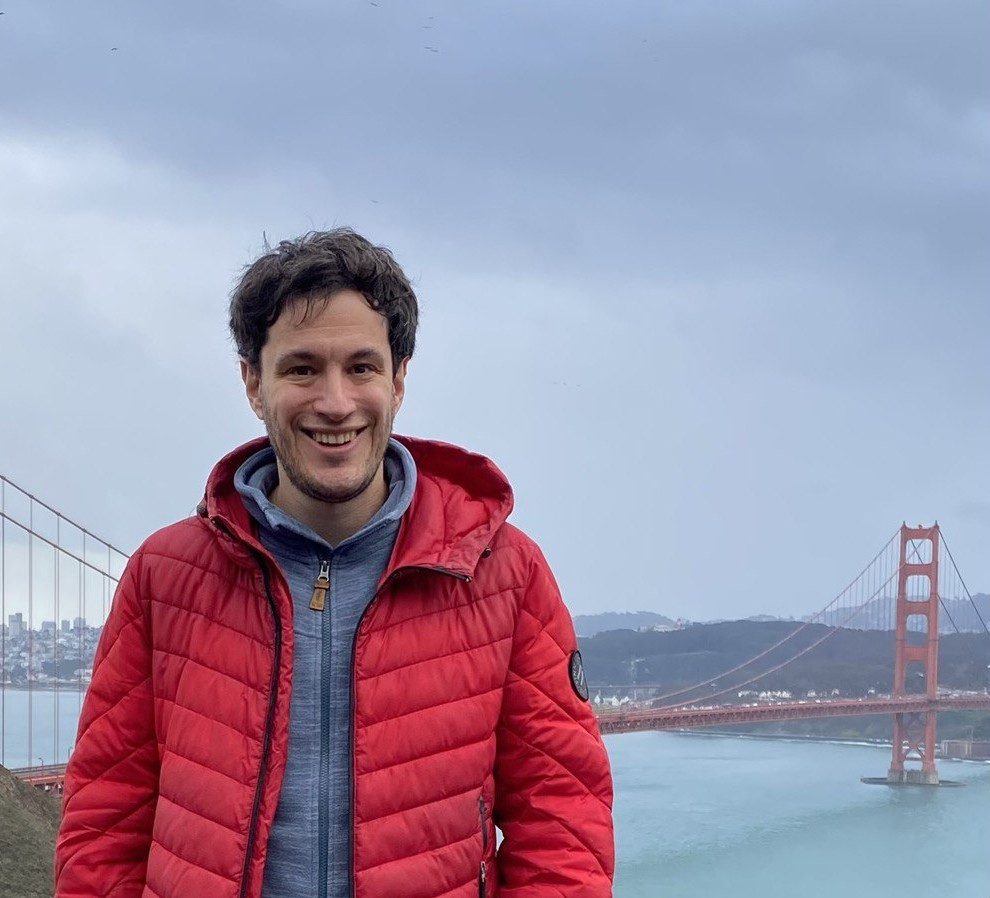# Oleg Butkovsky• Address: Mohrenstrasse 39, 10117 Berlin, Germany.
• Office: Mohrenstrasse 39, room 201.
• Phone: +49 30 20372-526.
• e-mail: oleg.butkovsky (at) wias (dash) berlin (dot) de.
• My CV and research statement.
• Most of my papers are available on arXiv.

I am a researcher in the Stochastic Algorithms and Nonparametric Statistics research group at the Weierstrass Institute for Applied Analysis and Stochastics.
Previously, I was a postdoc at Technion, Israel, where I worked with Leonid Mytnik, and at MSRI, California.
I got a PhD in Mathematics at Department of Mathematics of Moscow State University where I was working with Alexander Bulinski and Alexander Veretennikov.

I study how random noise changes the behavior of a complex deterministic system. More precisely, I investigate regularization–by–noise and stabilization–by–noise for ODEs and PDEs, ergodicity and stability for finite and infinite–dimensional Markov processes (stochastic PDEs, stochastic delay equations) as well as the long–term behavior of non–Markov processes (stochastic differential equations driven by fractional Brownian motion, McKean–Vlasov processes). I am also interested in construction of theoretically justified numerical algorithms related to the processes mentioned above.

Events:

## Preprints and Publications

### Regularization by noise for SDEs and SPDEs

1. O. Butkovsky, L. Mytnik (2019). Regularization by noise and flows of solutions for a stochastic heat equation. Annals of Probability, 47(1), 165–212.
2. S. Athreya, O. Butkovsky, L. Mytnik (2020). Strong existence and uniqueness for stable stochastic differential equations with distributional drift. Annals of Probability, 48(1), 178–210.
3. S. Athreya, O. Butkovsky, K. Lê, L. Mytnik (2020). Well-posedness of stochastic heat equation with distributional drift and skew stochastic heat equation. arXiv preprint arXiv:2011.13498, to appear in Communications on Pure and Applied Mathematics.
4. O. Butkovsky, K. Lê, L. Mytnik (2023). Stochastic equations with singular drift driven by fractional Brownian motion. arXiv preprint arXiv:2302.11937.

### Stabilization by noise and ergodicity

1. O. Butkovsky, A.Yu. Veretennikov (2013). On asymptotics for Wasserstein coupling of a Markov chain. Stochastic Processes and their Applications, 123(9), 3518–3541.
2. O. Butkovsky (2014). Subgeometric rates of convergence of Markov processes in the Wasserstein metric. Annals of Applied Probability, 24(2), 526–552.
3. O. Butkovsky, M. Scheutzow (2017). Invariant measures for stochastic functional differential equations. Electronic Journal of Probability, 22(98), 1–23.
4. O. Butkovsky, F. Wunderlich (2019). Asymptotic strong Feller property and local weak irreducibility via generalized couplings. arXiv preprint arXiv:1912.06121.
5. O. Butkovsky, A. Kulik, M. Scheutzow (2020). Generalized couplings and ergodic rates for SPDEs and other Markov models. Annals of Applied Probability, 30(1), 1–39.
6. O. Butkovsky, M. Scheutzow (2020). Couplings via comparison principle and exponential ergodicity of SPDEs in the hypoelliptic setting. Communications in Mathematical Physics, 379(3), 1001–1034.

### McKean–Vlasov equations and appications to finance

1. O. Butkovsky (2012) On the convergence of nonlinear Markov chains. Doklady Mathematics, 86(3), 824–826.
2. O. Butkovsky (2014) On ergodic properties of stochastic McKean–Vlasov equations. Theory of Probability and Its Applications, 58(4), 661–674.
3. C. Bayer, D. Belomestny, O. Butkovsky, J. Schoenmakers (2022). RKHS regularization of singular local stochastic volatility McKean-Vlasov models. arXiv preprint arXiv:2203.01160.

### Numerical methods for SDEs and SPDEs

1. O. Butkovsky, K. Dareiotis, M. Gerencsér (2021). Approximation of SDEs: a stochastic sewing approach. Probability Theory and Related Fields, 181(4), 975–1034.
2. O. Butkovsky, K. Dareiotis, M. Gerencsér (2022). Strong rate of convergence of the Euler scheme for SDEs with irregular drift driven by Levy noise. arXiv:2204.12926.
3. O. Butkovsky, K. Dareiotis, M. Gerencsér (2023). Optimal rate of convergence for approximations of SPDEs with non-regular drift. SIAM Journal on Numerical Analysis, 61(2), 1103–1137.

### Further applications of ergodic theory

1. O. Butkovsky, V. Margarint, Y. Yuan (2021). Law of the SLE tip. arXiv:2110.11247.